Do you like the article?
Share it with others —
Use new possibilities of MetaTrader 5

#### Similar articles# Combinatorics and probability theory for trading (Part III): The first mathematical model10 5152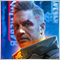### Introduction

In the previous article I obtained the first formula for fractals. This enabled the assumption that all important fractal-related values can be described using a mathematical model. The simulation is not intended to describe such values, while it only helped with the collection of the first data to enable a deeper analysis of such structures. In this article, I decided to pay special attention to describing the entire process of developing the first mathematical model, up to receiving the mathematical model which can be applied in different Expert Advisors.

### New ideas

When considering a symmetric fractal in the previous article, we obtained a general formula for calculating the average number of steps made by the price as a result of movement within a certain corridor, which is determined by the number of the same symmetric, smaller corridors. This formula is as follows:

1. S = K^2 - the average number of steps of the new corridor, based on the fact that a step is another corridor
2. P = K * P0  --> K = P/P0 - how many times the known corridor is larger than the unknown one
3. P - the width of the corridor whose average number of steps is not known (steps are half of the size of the original corridor)
4. P0 - the width of the known corridor

In order to be able to describe an asymmetric corridor, we need to redefine some of the previously mentioned concepts to make them more understandable. The most important of them is K. This value actually reflects the number of steps which the price should make in the new corridor, provided that the steps are only made up or only down. For a symmetric corridor, the number of steps will be equal regardless of which border we are considering (crossing), upper or lower. This is because the problem is mirrored both for the upper and the lower border. As for the asymmetry, as a result of code experimenting in the previous article, we obtained that the average number of steps for his case is determined as follows:

• S = n * m - average number of steps for asymmetric halves of the corridor
• n - the number of steps that fit in the upper half of the corridor
• m - the number of steps that fit in the lower half of the corridor

Hence, for the symmetric corridor we have "m = n". Then, based on the above:

• S = n * n = m * m = m * n = n^2 = m^2 = K^2
• K = n = m

It means that the first derived formula is only a special case of the previous one and thus K is no longer needed.

An interesting feature of this function is that S(a*k, b*k) = S(a,b) * S(k,k), which is very easy to prove:

• S(n*k ,m*b) = m*k*n*b = n*m * k*b
• S(n ,m) * S(k ,b) = n*m * k*b

This property is very interesting. With additional reflections, it can provide the derivation of all the necessary formulas to describe the entire fractal. This property reflects a very important property of any fractal: the capability of nesting. In other words, any finite fractal of great complexity can be represented as two or more simpler fractals which serve as steps in each other. This property will be used to generalize the formula for more complex cases.

Before proceeding with the fractals, let me remind you that the above defined formulas only work if p=0.5. As soon as the market or fractal parameters begin to deviate from a random walk, this formula begins to change very interestingly, namely:

• Ss[n,m,p] – a more general formula for the average number of steps (in any direction)
• S(n ,m)  = Ss[n,m,0,5] – formula for a random walk is a special case of the general formula

### General formula for the average number of steps

In order to determine the form of a more general formula, I used the invariance of the fractal nesting principle. If we consider any individual fractal nesting level relative to the previous one, there is no need to think about which steps occurred in a particular step. Thus, the primary up and down steps occur with precisely the same ratios of frequencies that corresponded to the situation of the previous level. In other words, crossing of a border of the corresponding nesting level is either a step up or a step down for the next nesting level. But it is also known that the ratio of the frequency of occurrence of steps for the current nesting level will not depend on the configuration of the current level. It means that the fractal nesting principle is observed for any probability “p”. It means that when the “p” value changes, the formula should also change but it should somehow preserve its nesting property. One simple experience can assist us in determining the general formula. We know that the probability p has a random walk point and two extreme points with zero and one. Let us view what values the function will take at these three points. So, we get the following:

1.  Ss[n,m,1] = Sn[n] = n
2. Ss[n,m,0] = Sm[m] = m
3.  Ss[n,m,0.5] = Sn[n] * Sm[m] = m*n
4.  Sn[n,p] – the number of steps in one direction till the upper border
5. Sn[m,p] – the number of steps in one direction till the lower border

In the first case we have no steps down — all chains follow the same route. The second case is opposite, and all steps will be downward, with no steps up. At extreme values, one of the factors completely disappears from the formula. It is possible if you raise it to zero. Any number to the power of zero is equal to 1. In addition, the degree has an invariance of the following form:

• A^X * B^X = (A*B)^X

If we substitute average steps instead of the numbers, the fractal nesting principle will still be preserved. Also, this will show that the power does not depend on n and m. The resulting general formula for the average number of steps is as follows:

• Ss[m,n,p] = ( Sn[n] ^ Pn[p] ) * ( S[m] ^ Pm[p] ) = (n ^ Pn[p] ) * ( m ^ Pm[p] )

The fractal nesting principle can be schematically shows like this: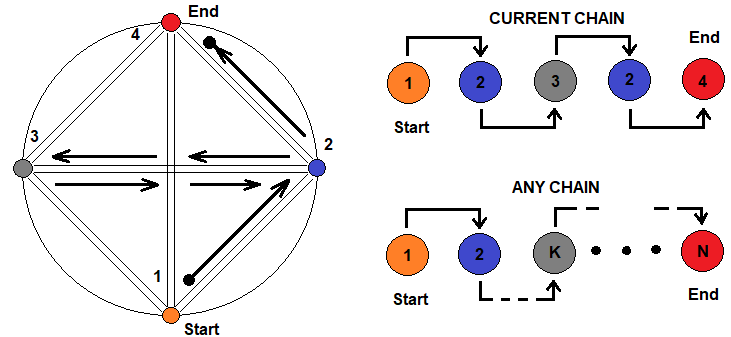The figure shows four states that symbolize different fractals which can be expressed by each other. The transition from one state to another is possible through any chain. An arbitrarily chosen chain is shown on the right. A little below it is shown that this chain can be of any length and complexity, and you can iterate through the same state an unlimited number of times. It means that the formula for the average number of steps in a fractal can be presented as a chain of products, which represent fractal nesting levels.

### Building a prototype of a power function

Further ideas come from the fractal nesting property and from the invariance of equal-power power functions relative to the base. All these mathematical principles will help derive further formulas for a deeper description of the fractals. Thus, we additionally get two functions, the form of which we don't know:

• Pn[p] – power for the upper border multiplier
• Pm[p] – power for the lower border multiplier

We know the following:

• Pn[0.5] = 1 , Pn = 1 , Pn = 0
• Pm[0.5] = 1 , Pm = 1 , Pm = 0

By analyzing power functions, it is possible to build a suitable function prototype. I've selected the following prototype:

1. Pn[p] = 1 , if p >= 0.5
2. Pn[p] = ( (1 – p)/0.5 ) ^ K
3. Pm[p] = 1 , if p <= 0.5
4. Pm[p] = ( p/0.5 ) ^ K
5. K is the power regulating the flatness of the function

It would be more accurate to represent “2” and “4” in the form of polynomials that can accurately describe the smooth transition of a given power, but I think that this is redundant here. If you wish to define more precise polynomials, here are the prototypes:

• Pn[p] = C1 * ( (1 – p)/0.5 ) ^ K1 + C2 * ( (1 – p)/0.5 ) ^ K2 … + … + CN * ( (1 – p)/0.5 ) *KN
• Pm[p] = C1 * ( p/0.5 ) ^ K1 + C2 * ( p/0.5 ) ^ K2 … + … + CN * ( p/0.5 ) ^ KN
• С1 + С2 + … + … СN = 1 are the wights for the relevant power
• KN is the power of the corresponding term

The polynomial that I have chosen is the simplest version of the general one with only one term. All declared principles can be checked for any fractional "n, m".

I have created the following program to check the above assumptions: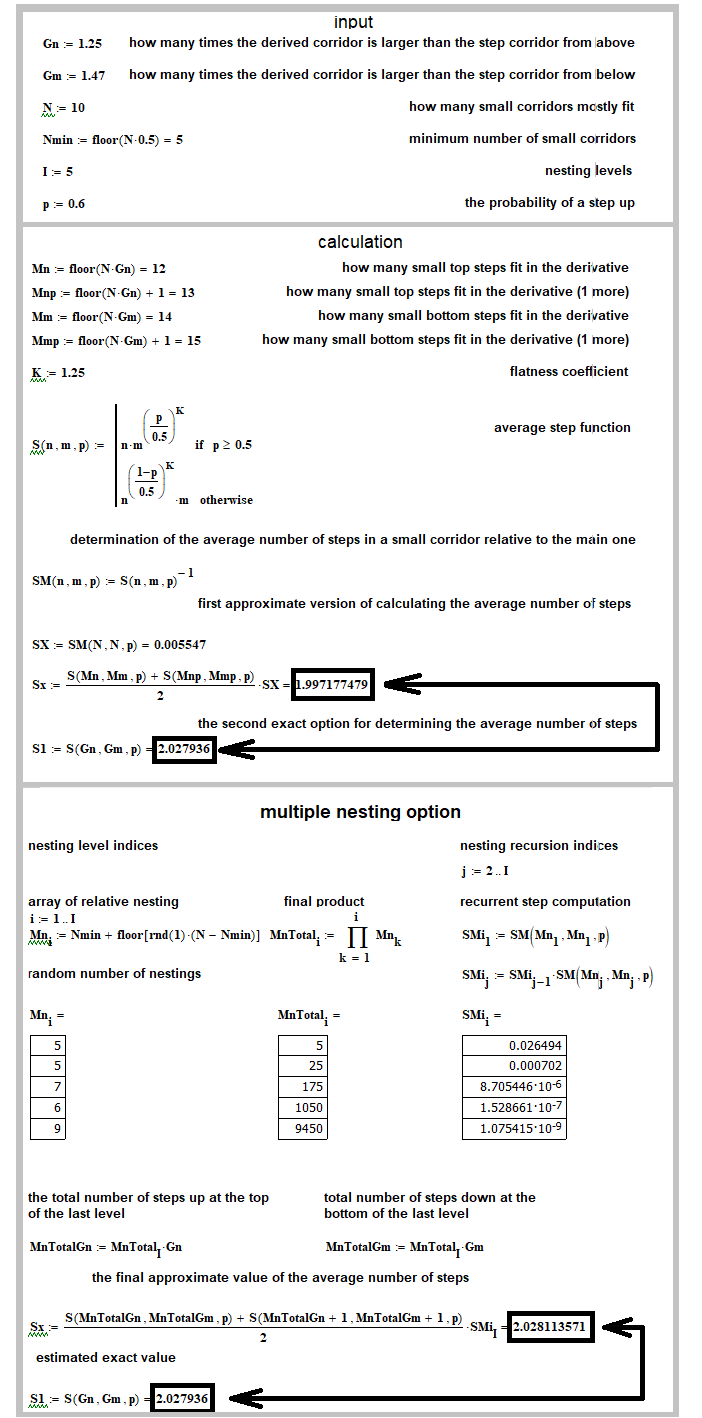As can be seen from the program results, it all  works as it should. To make sure, simply compare two numbers. If they are equal, then the nesting principle and the capability of using fractional n and m are confirmed.

### The general scheme of receiving the best function from the prototype-limited family

Now we need to determine how we will search for the required coefficients for the prototype. I've chosen the simplest search type – generating random numbers in the desired range. The method scheme is as follows: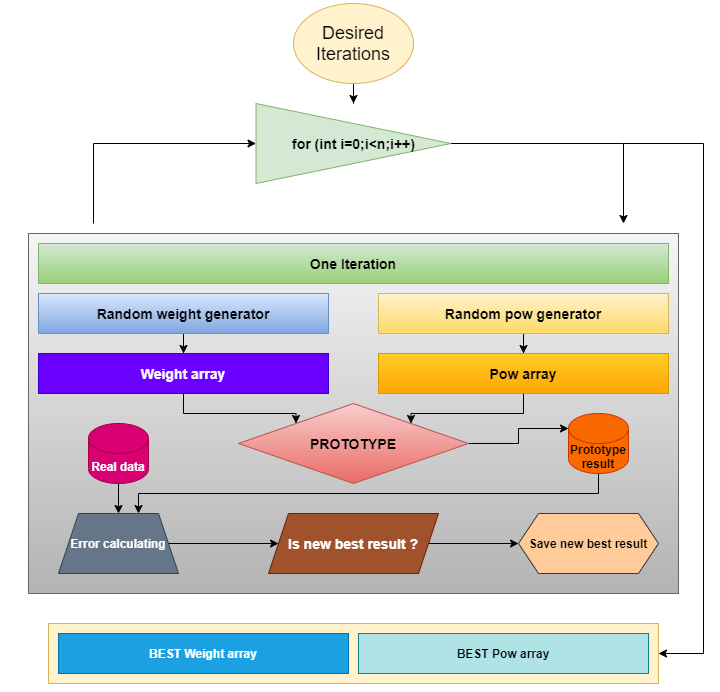It is suitable for any function prototype if we need to find coefficients for the best match of real data with the mathematical model. The same scheme will be valid further, as at the end of the article we will apply the same method to process another prototype.

### Deep analysis

Now let's take a deeper analysis of the structure of this formula. The result of the formula is the average number of steps which the price makes before it crosses one of the borders. Steps up and down are considered equal, but this equality is actually possible only in the case of a random walk. For a complete description of an asymmetric fractal, it is necessary to additionally determine what the given value actually consists of. Logically, the average number of any type of steps depends both on the average number of steps when crossing the upper border and the average number of steps when crossing the lower border. Let's find the relevant values for the upper border crossing:

• SP[U,ud] = S[U,u] * P[U] + S[U,d] * P[U] = (S[U,u] + S[U,d]) * P[U] = S[U] * P[U]
• S[U] – the average number of any steps provided that the chain of steps first reaches the upper border
• S[U,u] – the average number of steps up provided that the chain of steps first reaches the upper border
• S[U,d] – the average number of steps down provided that the chain of steps first reaches the upper border
• P[U] – the probability that the price will first cross the upper border
• SP[U,ud] – the average probable number of steps to cross the upper border

The formulas are similar for the lower border:

• SP[D,ud] = S[D,u] * P[D] + S[D,d] * P[D] = (S[D,u] + S[D,d]) * P[D] = S[D] * P[D]
• S[D] – the average number of any steps provided that the chain of steps first reaches the lower border
• S[D,u] – the average number of steps up provided that the chain of steps first reaches the lower border
• S[D,d] – the average number of steps down provided that the chain of steps first reaches the lower border
• P[D] – the probability that the price will first cross the lower border
• SP[D,ud] – the average probable number of steps to cross the lower border

It becomes clear that:

• S = SP[U,ud] + SP[D,ud]

Ultimately, all the values that we can finally obtain and use, depend on five fundamental values:

• S[U,u] = SP[U,u]/P[U] – the average number of steps up provided that the chain of steps first reaches the upper border
• S[U,d] = SP[U,d]/P[U] – the average number of steps down provided that the chain of steps first reaches the upper border
• S[D,u] = SP[D,u]/P[D] – the average number of steps up provided that the chain of steps first reaches the lower border
• S[D,d] = SP[D,d]/P[D] – the average number of steps down provided that the chain of steps first reaches the lower border
• P[U] – the probability that the price will first cross the upper border

We will search for the formulas for these values based on the results of fractal operation with different input parameters. In the previous article, I selected the following values for the fractal output:

• SP[U,u] – the average number of steps up provided that the chain of steps first reaches the upper border
• SP[U,d] – the average number of steps down provided that the chain of steps first reaches the upper border
• SP[D,u] – the average number of steps up provided that the chain of steps first reaches the lower border
• SP[D,d] – the average number of steps down provided that the chain of steps first reaches the lower border
• P[U] – the probability that the price will first cross the upper border
• P[D] – the probability that the price will first cross the lower border
• S = SP – the average number of any steps

Here is a separate group of elements that can be expressed in terms of fundamental values:

• SP[U]
• S[U,ud] = S[U,u] + S[U,d]
• S[D,ud] = S[D,u] + S[D,d]
• P[D] = 1 – P[U]
• S = SP[U,ud] + SP[D,ud]

While dealing with the mathematics of fractals, I performed extensive analysis, which can be briefly shown in a compact diagram. The diagram shows the resulting process of searching for a mathematical model: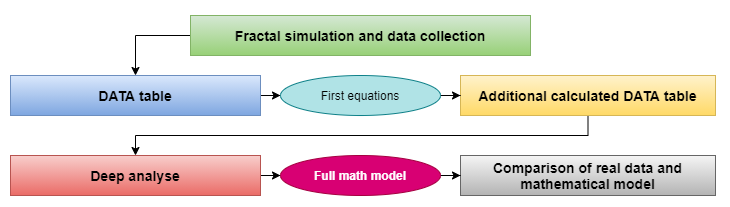### Data collection and conclusions

As a result of a more detailed consideration of fractal calculation results, I noticed that the six values, which were initially determined when constructing the concept of a universal fractal, are mathematically related. Initially I conducted tests for symmetric borders and tried to identify dependencies between these values. I got some results. I did the calculations for ten different cases, and it turned out to be enough:

 Test index Steps in the upper half of the corridor (n) Steps in the lower half of the corridor ( m ) Probability of the initial step   ( p ) Average probable number of up steps for the upper border ( SP[U,u]  ) Average probable number of down steps for the upper border ( SP[U,d]  ) Average probable number of up steps for the lower border ( SP[D,u]  ) Average probable number of down steps for the lower border ( SP[D,d]  ) Average probable number of any steps for the upper border ( SP[U,ud]  ) Average probable number of any steps for the lower border ( SP[D,ud]  ) 1 1 1 0.5 0.5 0 0.0 0.5 0.5 0.5 2 2 2 0.5 1.5 0.5 0.5 1.5 2.0 2.0 3 3 3 0.5 3.0 1.5 3.0 1.5 4.5 4.5 4 1 2 0.5 0.888888 0.2222222 0.111111 0.777777 1.11111111 0.8888888 5 2 3 0.5 2.2 1.0 0.8 2.0 3.2 2.8 6 1 2 0.6 1.038781 0.249307 0.066481 0.487534 1.288088 0.554016 7 2 3 0.6 2.811405 1.191072 0.338217 0.906713 4.0024777 1.244931 8 2 3 1.0 2.0 0.0 0.0 0.0 2.0 0.0 9 1 3 0.5 1.25 0.5 0.25 1 1.75 1.25 10 1 4 0.5 1.6 0.8 0.4 1.2 2.4 1.6

Below I will show a table with calculated values which are not displayed in the fractal log. We will need them to evaluate the dependencies between values:

 Test index Steps in the upper half of the corridor (n) Steps in the lower half of the corridor ( m ) Upper border crossing probability ( P(U) ) Lower border crossing probability ( P(D) ) Average number of any steps when crossing the upper border (S[U,ud]) = SP[U,ud]/P[U] Average number of any steps when crossing the lower border (S[D,ud]) = SP[D,ud]/P[D] Average number of up steps for the upper border ( S[U,u]  ) = SP[U,u]/P[U] Average number of down steps for the upper border ( S[U,d]  ) = SP[U,d]/P[U] Average number of up steps for the lower border ( S[D,u]  ) = SP[D,u]/(P[D]) Average number of down steps for the lower border ( S[D,d]  ) = SP[D,d]/(P[D]) Average number of steps   ( S ) 1 1 1 0.5 0.5 1.0 1.0 1.0 0.0 0.0 1.0 1 2 2 2 0.5 0.5 4.0 4.0 3.0 1 1 3 4 3 3 3 0.5 0.5 9.0 9.0 6 3 3 6 9 4 1 2 0.66666 0.3333333 1.6666666 2.6666666 1.3333333 0.33333333 0.33333333 2.33333333 2 5 2 3 0.6 0.4 5.3333333 7 3.6666666 1.66666666 2 5 6 6 1 2 0.789473 0.210526 1.631579 2.631579 1.315790 0.315789 0.315789 2.315789 1.842104 7 2 3 0.810166 0.189498 4.940318 6.569626 3.470159 1.470157 1.784805 4.784 5.2474087 8 2 3 1.0 0.0 2.0 0.0 2.0 0.0 0.0 0.0 2.0 9 1 3 0.75 0.25 2.3333333 5 1.6666666 0.6666666 1 4 3 10 1 4 0.8 0.2 3.0 8.0 2 1 2 6 4

From this table, you can find the first two equations that are needed to calculate all four unknown values (of which all other values are composed). To get the formulas, pay attention to columns S[U,u], S[U,d], S[D,u], S[D,d]. It is very interesting that the numbers in this column have the same fractional part pairwise in columns S[U,u], S[U,d] and in S[D,u], S[D,d]. Also, you can see that S[U,u] > S[U,d] and S[D,d] > S[D,u]. If we subtract these values in pairs and compare with m, n, then it turns out that this difference is exactly equal to the corresponding number of steps to the upper or lower border:

• S[U,u] – S[U,d] = n
• S[D,d] – S[D,u] = m

Thus, we get two very important values that will help determine the fundamental values. Two equations are not enough. But we can get two more equations, which will allow the determining of the same values based on a slightly different logic. If we experiment with the fractal for an infinitely long time, then the ratio of frequency of occurrence of up and down steps will be proportionate to the corresponding probabilities.

These ratios can be obtained if we assume that:

1. Lim[N0 -- > +infinity] (N0[U]/N0) = p
2. Lim[N0 -- > +infinity] (N0[D]/N0) = 1-p
3. Lim[N0 -- > +infinity] (N0[U]/N) = S[UD,u]
4. Lim[N0 -- > +infinity] (N0[D]/N) = S[UD,d]
5. Lim[N0 -- > +infinity] (N0/N) = S
6. N0 – the number of elementary experiments performed in relation to initial steps (make up a complex experiment)
7. N – the number of complex experiments that are made up of simple experiments

If we introduce the multiplier N0/N0 = 1 into “3” and “4” and properly arrange the fractions inside, then we get the following:

• Lim[N0 -- > +infinity] (N0[U]/N) = Lim[N0 -- > +infinity] (N0[U]/N0 * N0/N) = Lim[N0 -- > +infinity] (N0[U]/N0)  * Lim[N0 -- > +infinity] (N0/N) =  p*S  = S[UD,u]
• Lim[N0 -- > +infinity] (N0[D]/N) = Lim[N0 -- > +infinity] (N0[D]/N0 * N0/N) = Lim[N0 -- > +infinity] (N0[D]/N0)  * Lim[N0 -- > +infinity] (N0/N) = (p-1)*S  = S[UD,d]

The product limit can be represented as the product of the limits, if each of these limits is a finite number. Our limits comply with this condition. This is how these formulas are derived. The formulas are as follows:

• S[UD,u] = S*p
• S[UD,d] = S*(1-p)

It is better to express these values in terms of fundamental values — this provides two missing equations to determine everything we need:

• S[U,u] * P[U] + S[D,u] * ( 1 – P[U] ) = S*p
• S[U,d] * P[U] + S[D,d] * ( 1 – P[U] ) = S*(1-p)

As a result, four equations have five unknown values. The fifth unknown is one of the probabilities that form the full group (probability of reaching one of the borders). In order to be able to find all five unknowns, we need the fifth equation, because a system of equations can have a unique solution only if the number of equations is equal to the number of unknowns. The fifth equation can be obtained intuitively, as we know that it is the difference between the up and down steps. Ideally, it is the limit:

• Lim[Nt[U] -- > +infinity] ((N0[U] - N0[D])/(Nt[U] - Nt[D]) = 1
• Nt[U] =  – the ideal number of basic steps up, calculated using a probability of a basic step up
• Nt[D] – the ideal number of basic steps down, calculated using a probability of a basic step down
• N0[U] – real number of basic steps up
• N0[D] – real number of basic steps down

We can find a similar limit using the border crossing probabilities:

• Lim[Nb[U] -- > +infinity] ((N0[U] - N0[D])/(Nb[U] - Nb[D]) = 1
• Nb[U] – the ideal number of basic steps up, calculated using a probability of crossing the upper border
• Nb[D] – the ideal number of basic steps down, calculated using a probability of crossing the lower border
• N0[U] – real number of basic steps up
• N0[D] – real number of basic steps down

Using these two limits, we can make up a more complex one, such as their sum, difference or quotient. I will choose the quotient, which will reduce the following expression and will completely eliminate the limit N0 [U] - N0 [D]. By dividing these two limits and by transforming the equation, we get the following:

• P[U] * n – (1 - P[U]) * m = p * S - (1 - p) *S

This is the fifth equations, from which we can find the probabilities of crossing the borders. As a result, we get a system of five equations. It will look like this:

1. S[U,u] – S[U,d] = n
2. S[D,d] – S[D,u] = m
3. S[U,u] * P[U] + S[D,u] * ( 1 – P[U] ) = S*p
4. S[U,d] * P[U] + S[D,d] * ( 1 – P[U] ) = S*(1-p)
5. P[U] * n – (1 - P[U]) * m = p * S - (1 - p) *S = 2*p*S - S

The initial system of equations with four unknowns also provides the same resulting equation.  The system can be solved in a classical way, by successively excluding the variables:

• -->  S[U,u] = n + S[U,d] – exclude “S[U,u]”
• -->  S[D,d] = m + S[D,u] – exclude “S[D,d]”
• (n + S[U,d]) * P[U] + S[D,u] * ( 1 – P[U] ) = S*p – substitute everything to equation 3
• S[U,d] * P[U] + (m + S[D,u]) * ( 1 – P[U] ) = S*(1-p) – substitute everything to equation 4

After these transformations, we only need to subtract equation 3 from 4, after which we get the same equation that we obtained intuitively. Unfortunately, this system of equations does not allow us to find the four remaining values. I hoped this system would work but it didn't. To understand the reason, I had to analyze the table with fractal data. This enabled me to create a formula for one of these four quantities. With this additional formula, we can find all the rest values. So, the system turned out to be useful.

### Algorithm for calculating the entire mathematical model

First, let's define the inverse equations and the sequence of their use in order to find all the other, knowing S[U,u]. I use this value because I managed to find the relevant formulas for its calculation. With this value, we can immediately find S[U,d] using the first equation:

• S[U,d] = S[U,u] – n

Then, substitute these two values into equations 3 and 4, and find the remaining values S[D,u] and S[D,d]. S[D,u] can be calculated immediately from the third equation:

• S[D,u] = ( S*p – S[U,u] * P[U] ) / ( 1 – P[U] )

Now, we only need to find a formula for the last unknown value. Substitute the obtained expression for S[U,d] into the fourth equation:

• S[D,d] = ( S*(1-p) - S[U,d] * P[U]) / ( 1 – P[U] ) =  ( S*(1-p) - ( S[U,u] – n ) * P[U] ) / ( 1 – P[U] )

The only missing element is the P[U] value which can be easily obtained by solving the fifth equation. Let's do this:

• P[U] * n – (1 - P[U]) * m = 2*p*S – S
• --> P[U] * (n + m)  =   2 * p * S – S + m
• --> P[U] = ( 2 * p * S – S + m ) / (n + m)

The known values here are the following:

• n – the number of steps up till the upper border
• m – the number of steps down till the lower border
• p – the probability of the initial step up
• S[U,u] – the average number of steps up provided that the upper border is crossed
• P[U] – the probability of crossing the upper border

Value 4 can be calculated:

• S[U,u] = Ss[m,n,p] = (n ^ Pn[p] ) * ( m ^ Pm[p] )
• Pn[p] = 1 , if p >= 0.5
• Pn[p] = ( (1 – p)/0.5 ) ^ K
• Pm[p] = 1 , if p <= 0.5
• Pm[p] = ( p/0.5 ) ^ K
• K is the power regulating the flatness of the function

We will calculate the flatness coefficient in a separate program a little later. Now we need to determine the most important value. By analyzing value S[U,u] from the table, I managed to derive formulas for two values for symmetric borders:

1. S[U,u] = Summ[ i = 0, n] ( i ) ; if n == m
2. S[U,d] = Summ[ i = 0, n] ( i-1 ) ; if n == m

The problem is that these formulas only work for p = 0.5 and symmetric borders. The concept of the formulas should be expanded to cover asymmetric borders. After that, we can generalize it for different p values. Before we proceed to the generalization, pay attention that test results in the table are only applicable for “m > n”. If “m < n”, formulas will work for “S[D,d], S[D,u]”. In this case, it is necessary to create a mirror analogue of the algorithm for finding all other unknown values.

Similarly, let's define the inverse equations and the order in which they should be used for the case of S[D,d]. Again, find value S[D,u] using the second equation:

• S[D,u] = S[D,d] – m

After that both values can be substituted into equations 3 and 4 in order to find S[U,u] and S[U,d]. S[D,u] can be calculated immediately from the fourth equation:

• S[U,d] = ( S*(1-p) - S[D,d] * ( 1 – P[U] ) ) / P[U]

Now, we only need to find a formula for the last unknown value. Substitute the obtained expression for S[U,d] into the third equation:

• S[U,u] = ( S*p – ( S[D,d] – m ) * ( 1 – P[U] ) ) / P[U]

As a result, we obtained all the required data to generalize the formulas S[U,u], S[D,d] for cases where "n != m". The following data were obtained from the analysis of table data:

1. m > n, p = 0,5
2. S[U,u] = Summ[ i = 0 … n] ( i ) + (m-1)/3

For the opposite case:

1. m < n, p = 0,5
2. S[D,d] = Summ[ i = 0 … m] ( i ) + (n-1)/3

The calculations will be simpler for the standard case with symmetric borders:

1. m = n, p = 0,5
2. S[U,u] = Summ[ i = 0 … n] ( i )
3. S[D,d] = Summ[ i = 0 … m] ( i )

### Prototypes for getting the last equation

Now let's define a prototype of the modified function that will describe S[U,u], S[D,d] for all possible p values. To build a basic working prototype, we need three points on the p-axis and a few assumptions regarding the overall structure. I believe it is sufficient to consider two types of generic function:

1. Sp[U,u] = S[U,u] ^ K(p)
2. Sp[D,d] = S[D,d] ^ K(q)
3. = 1-p

The first type can be a real working prototype or a certain marker indicating that the structure is different and requires another logic. It turned out that the power function is capable to bring all the data together. Of course, there can be more complex prototypes, perhaps more accurate, but I think our solution is quite sufficient. The most important thing is to understand the logic. If, however, you want to fine tune the model, you will be able to do so based on the data presented in the article. I have created a test program: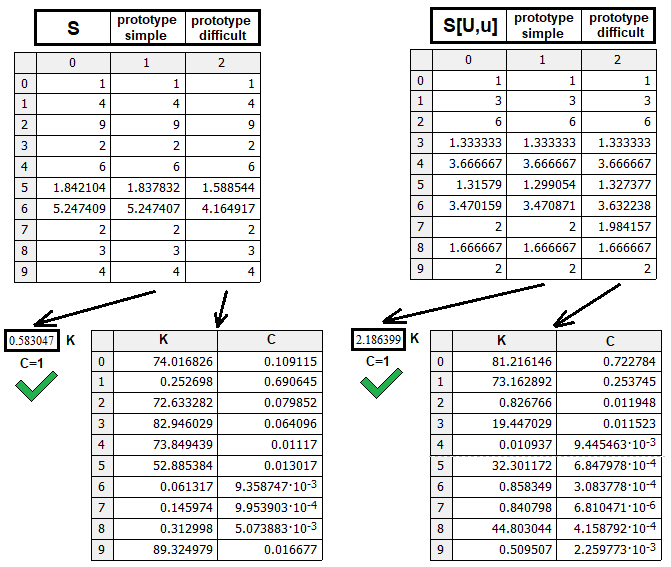The program checks both prototypes, S(n,m,p) and S[U,u](n,m,p). No checking is needed for S[D,d](n,m,p), as this function is mirrored to S[U,u](n,m,p), and thus S[D,d](n,m,p) = S[U,u](m,n,p-1). The figure shows the comparison of found prototypes in terms of their efficiency. Each prototype was tested with the same number of random combinations of weights and power coefficients in the formulas. The simpler prototype shows a more beautiful results with the same number of search cycles. If necessary, it is possible to conduct additional calculations to see what more complex prototypes are capable of.

After checking, we need to define the internal structure of the nested function “K(p)”, “K(q)”. Its internal structure must provide the obligatory coincidence in points p=0, p=0.5, p=1, q=0, q=0.5, q=1. We know the function values in these points, which enables easier selection of the required prototype:

1. p = 0.5 ; Sp[U,u] = S[U,u] --> K(0.5) = 1 ,
2. p = 1.0 ; Sp[U,u] = n = S[U,u]^(Log[S[U,u]-->n]) --> K(1.0) = Log[S[U,u]-->n]
3. p = 0.0 ; Sp[U,u] = 0 = S[U,u]^(-infinity) --> K(0.0) = -infinity
4. q = 0.5 ; Sp[D,d] = S[D,d] --> K(0.5) = 1 ,
5. q = 1.0 ; Sp[D,d]  = n = S[D,d]^(Log[S[U,u]-->m]) --> K(1.0) = Log[S[D,d]-->m]
6. q = 0.0 ; Sp[D,d] = 0 = S[D,d]^(-infinity) --> K(0.0) = -infinity

The first and the fourth expressions show that the power should be equal to one at the random walk point. The second and the fifth expressions indicate that the power should be such that, when a value is raised to it, we get either “n” or “m”, which can be seen in the results table above. The third and the sixth expressions show that the power should tend to minus infinity to provide zero. This fact additionally implies that the values p and q should be in the denominator of the prototype, as division by zero leads to such values as infinity. We already had experience building a power-law prototype for a function. We can use it as a basis and revise. After an in-depth analysis of the problem, I came to this prototype:

• K(p) = 1 + D * Summ(N) (Kn *  | (( p – 0.5 )/(0.5*p)) | ^ A  + Kn * | (( p – 0.5 )/(0.5*p)) | ^ A + …. + Kn[N] * | (( p – 0.5 )/(0.5*p)) | ^ A[N])
• Kn + Kn + …. Kn[N] = Log[S[U,u]-->n] – 1
• D = (( p – 0.5 )/(0.5*p)) / | (( p – 0.5 )/(0.5*p)) |
• K(q) = 1 + C * Summ(N) (Km * (( q – 0.5 )/(0.5*q)) ^ B  + Km * (( q – 0.5 )/(0.5*q)) ^ B + …. + Km[N] * (( q – 0.5 )/(0.5*q)) ^ B[N])
• Km + Km + …. Km[N] = Log[S[D,d]-->m] – 1
• C = (( q – 0.5 )/(0.5*q)) / | (( q – 0.5 )/(0.5*q)) |
• Kn, Km – weights of the appropriate terms
• A, B - sensitivity of terms

The function looks complicated, but it is based on simple logic. If “p=0.5, q=0.5”, everything under the sum sign turns to zero and only 1 remains, thus providing conditions “1”, “4”. If “p=1, q=1”, then the relevant fractions within the sum, which are raised to a power, turn to 1: |(( p – 0.5 )/(0.5*p)) |. These terms are written with the modulus sign to exclude complex values of the function when raising to a power. Instead, the relevant sign is provided as an additional factor. The power no longer affects these terms, and the entire sum turns to Log[S[U,u]-->n] – 1, Log[S[D,D]-->m] - 1. By adding this number to 1 we obtain the required values of the function: Log[S[U,u]-->n], Log[S[D,d]-->m].

Interpolation polynomials are constructed according to a similar logic, an example of which is the well-known Lagrange polynomial. Our polynomial is designed for a specific task, for which it can be applied. Its advantage is the full adaptivity to the task. In order to find the desired function from this family of curves, we only need to find two arrays of numbers.

### Mathematical model implementation and testing

With the found expressions, we can easily implement the necessary functionality to calculate any fractal. We will need only one structure which will be a container for all data that the main function will return. Other functions will be auxiliary. We need only the last function:

```struct MathModel1//structure for the first mathematical model
{
double S;//the average number of any steps
double pU;//the probability that the price will first reach the upper border
double pD;//the probability that the price will first reach the lower border
double SUu;//the average number of steps up if the price first reaches the upper border
double SUd;//the average number of steps down if the price first reaches the upper border
double SDu;//the average number of steps up if the price first reaches the lower border
double SDd;//the average number of steps down if the price first reaches the lower border

double SPUu;//the average probable number of steps up if the price first reaches the upper border
double SPUd;//the average probable number of steps down if the price first reaches the upper border
double SPDu;//the average probable number of steps up if the price first reaches the lower border
double SPDd;//the average probable number of steps down if the price first reaches the lower border

double SPUud;//the average probable number of steps in any direction if the price first reaches the upper border
double SPDud;//the average probable number of steps in any direction if the price first reaches the lower border

double SUDu;//the average number of steps up when reaching any of the borders
double SUDd;//the average number of steps down when reaching any of the borders
};

double Ss(int n, int m,double p, double K)//prototype of the function of the average number of steps in any direction when reaching any border
{
if (p>=0.5) return n*MathPow(m,MathPow((1-p)/0.5,K));
else return MathPow(n,MathPow(p/0.5,K))*m;
}

double Log(double a, double b)//logarithm function for any base
{
if (MathLog(a) != 0) return MathLog(b)/MathLog(a);
else return 0.0;
}

double SUu(int n,int m)//average number of steps up to the upper border if p=0.5
{
double rez=0.0;
if (m>n)
{
for (int i=0;i<=n;i++) rez+=double(i);
rez+=(m-1)/3.0;
}
if (m==n) for (int i=0;i<=n;i++) rez+=double(i);
return rez;
}

double SDd(int n,int m)//average number of steps down to the lower border if p=0.5
{
double rez=0.0;
if (n>m)
{
for (int i=0;i<=m;i++) rez+=double(i);
rez+=(n-1)/3.0;
}
if (m==n) for (int i=0;i<=m;i++) rez+=double(i);
return rez;
}

double KpnEasy(int n,int m, double p,double A)//power prototype for steps up m>=n
{
double D;
if ( p-0.5 != 0 ) D=(p-0.5)/MathAbs(p-0.5);
else D=1.0;
return 1.0 + D*(Log(SUu(n,m),n) - 1)*MathPow(((p-0.5)/(0.5*p)),A);
}

double KpmEasy(int n,int m,double p,double A)//power prototype for steps down m<n
{
double D;
if ( 0.5-p != 0 ) D=(0.5-p)/MathAbs(0.5-p);
else D=1.0;
return 1.0 + D*(Log(SDd(n,m),m) - 1)*MathPow(((0.5-p)/(0.5*(1.0-p))),A);
}

double SUuS(int n,int m,double p, double A)//full prototype for average steps up m>=n
{
return MathPow(SUu(n,m),KpnEasy(n,m,p,A));
}

double SDdS(int n,int m,double p, double A)//full prototype for average steps down  n>m
{
return MathPow(SDd(n,m),KpmEasy(n,m,p,A));
}

MathModel1 CalculateMathModel(int n, int m, double p,double K=0.582897,double A=2.189246)//calculating the entire mathematical model
{
MathModel1 Mt;
if ( m >= n )
{
Mt.S=Ss(n,m,p,K);
Mt.pU=(2*p*Mt.S-Mt.S+m)/(n+m);
Mt.pD=1.0-Mt.pU;
Mt.SUu=SUuS(n,m,p,A);
Mt.SUd=Mt.SUu-n;
if (1.0-Mt.pU != 0.0) Mt.SDu=(Mt.S*p-Mt.SUu*Mt.pU)/(1.0-Mt.pU);
else Mt.SDu=0.0;
if (1.0-Mt.pU != 0.0) Mt.SDd=(Mt.S*(1.0-p)-Mt.SUd*Mt.pU)/(1.0-Mt.pU);
else Mt.SDd=0.0;
}
else
{
Mt.S=Ss(n,m,p,K);
Mt.pU=(2*p*Mt.S-Mt.S+m)/(n+m);
Mt.pD=1.0-Mt.pU;
Mt.SDd=SDdS(n,m,p,A);
Mt.SDu=Mt.SDd-m;
if (Mt.pU != 0.0) Mt.SUd=(Mt.S*(1.0-p)-Mt.SDd*(1.0-Mt.pU))/Mt.pU;
else Mt.SUd=0.0;
if (Mt.pU != 0.0) Mt.SUu=(Mt.S*p-Mt.SDu*(1.0-Mt.pU))/Mt.pU;
else Mt.SUu=0.0;
}

Mt.SPUu=Mt.SUu*Mt.pU;
Mt.SPUd=Mt.SUd*Mt.pU;
Mt.SPDu=Mt.SDu*Mt.pD;
Mt.SPDd=Mt.SDd*Mt.pD;

Mt.SPUud=Mt.SPUu+Mt.SPUd;
Mt.SPDud=Mt.SPDu+Mt.SPDd;

Mt.SUDu=Mt.SPUu+Mt.SPDu;
Mt.SUDd=Mt.SPUd+Mt.SPDd;

return Mt;
}
```

To check the mathematical model, I implemented an analogue of this code in MathCad15. If the mathematical model is composed correctly, then the results from the table should coincide with the result returned by the mathematical model. The program listing is attached to the article, so you can check it yourself. I decided not to add show code directly in the article as it would take up too much space in the article, but you should definitely see the result. Let's compare the matrices and make sure that the mathematical model is workable: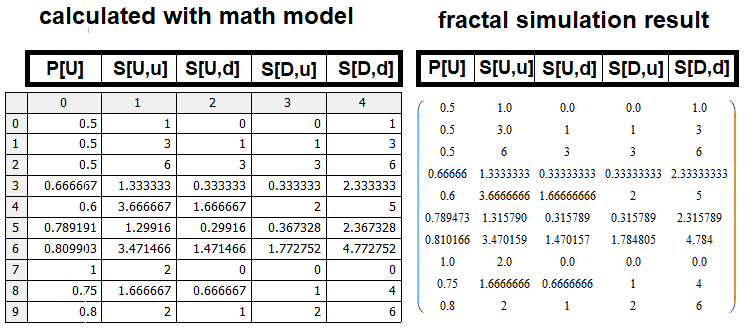Of course, there are some inaccuracies, which are though due to the efficiency to our found prototypes for the values S, S[U,u], S[D,d]. An additional factor for minor differences can be related to simulation inaccuracies, which are greater for larger values of simulated n, m, as we have to limit the simulation depth due to the limited computing power.

### Conclusion

I spent a lot of time developing this mathematical model, because I had to come up with all the mathematics from scratch. Anyway, I am happy with the result. In the next articles, I will try to develop more universal mathematical models which, when combined, would allow the calculation of any trading configuration in terms of its main characteristics. Furthermore, the mathematical model is suitable not only for describing pricing processes, but it can also be used for describing trading signals and for simplifying complex strategies, reducing them to simpler ones. This will take some time.

### References

• #### Combinatorics and probability theory for trading (Part I): The basics

Translated from Russian by MetaQuotes Ltd.
Original article: https://www.mql5.com/ru/articles/9570

Attached files |
Materials.zip (312.85 KB)

#### Other articles by this author

Last comments | Go to discussion (2)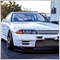| 6 Oct 2021 at 18:33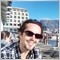| 8 Oct 2021 at 08:15
Incredible depth and consideration, im floored, not sure what you are trying to calculate, I read through most of it but its above my iq at this time in the morning, and im not paying full attention as i dont see what the goal is. We have fractals as an indicator, yes and you are trying to calculate what exactly. Great effort, really big round of applause.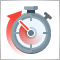Dealing with Time (Part 2): The Functions
Determing the broker offset and GMT automatically. Instead of asking the support of your broker, from whom you will probably receive an insufficient answer (who would be willing to explain a missing hour), we simply look ourselves how they time their prices in the weeks of the time changes — but not cumbersome by hand, we let a program do it — why do we have a PC after all.Dealing with Time (Part 1): The Basics
Functions and code snippets that simplify and clarify the handling of time, broker offset, and the changes to summer or winter time. Accurate timing may be a crucial element in trading. At the current hour, is the stock exchange in London or New York already open or not yet open, when does the trading time for Forex trading start and end? For a trader who trades manually and live, this is not a big problem.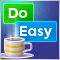Graphics in DoEasy library (Part 83): Class of the abstract standard graphical object
In this article, I will create the class of the abstract graphical object. This object is to serve as a basis for creating the class of standard graphical objects. Graphical objects feature multiple properties. Therefore, I will need to do a lot of preparatory work before actually creating the abstract graphical object class. This work includes setting the properties in the library enumerations.Graphics in DoEasy library (Part 82): Library objects refactoring and collection of graphical objects
In this article, I will improve all library objects by assigning a unique type to each object and continue the development of the library graphical objects collection class.